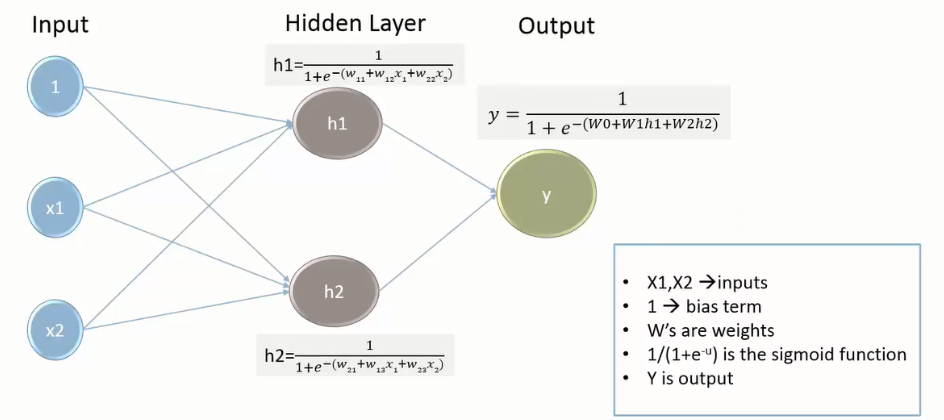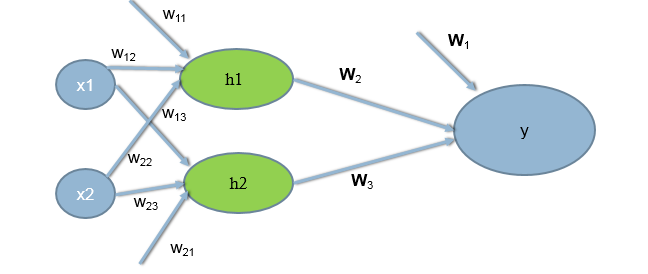• No products in the cart.

# 204.5.6 Neural Network Intuition

##### Neural network at a glance.

Link to the previous post : https://test.statinfer.com/204-5-5-practice-implementing-intermediate-outputs-in-python/

Before going further into neural network algorithm, we need to understand and break down how the algorithm is working.

### Neural Network Intuition

Final Output

y=out(h)=g(Wjhj)hj=out(x)=g(w(jk)xk)

y=out(h)=g(Wjg(w(jk)xk))

• So h is a non linear function of linear combination of inputs – A multiple logistic regression line.
• Y is a non linear function of linear combination of outputs of logistic regressions.
• Y is a non linear function of linear combination of non linear functions of linear combination of inputs.
• We find W to minimize ni=1[yig(Wjhj)]2
• We find  Wj and wjk to minimize ni=1[yig(Wjg(w(jk)xk))]2
• Neural networks is all about finding the sets of weights  Wj and wjk using Gradient Descent Method.

### The Neural Networks

• The neural networks methodology is similar to the intermediate output method explained above.
• But ,we will not manually subset the data to crate the different models.
• The neural network technique automatically takes care of all the intermediate outputs using hidden layers.
• It works very well for the data with non-linear decision boundaries.
• The intermediate output layer in the network is known as hidden layer.
• In Simple terms, neural networks are multi layer nonlinear regression model.
• If we have sufficient number of hidden layers, then we can estimate any complex non-linear function.

#### Neural Network and VocabularyWhy are they called hidden layers?

• A hidden layer “hides” the desired output.
• Instead of predicting the actual output using a single model, build multiple models to predict intermediate output
• There is no standard way of deciding the number of hidden layers.

#### Algorithm for Finding Weights• Algorithm is all about finding the weights/coefficients
• We randomly initialize some weights; Calculate the output by supplying training input; If there is an error the weights are adjusted to reduce this error.

The next post is about the neural network algorithm .

Link to the next post : https://test.statinfer.com/204-5-7-the-neural-network-algorithm/

21st June 2017

### 0 responses on "204.5.6 Neural Network Intuition"

Statinfer Software Solutions LLP

Software Technology Parks of India,
NH16, Krishna Nagar, Benz Circle,